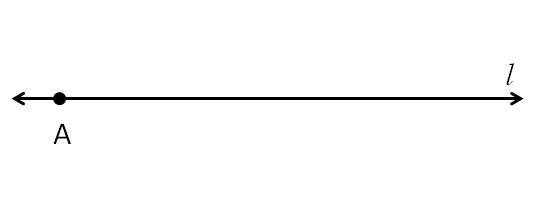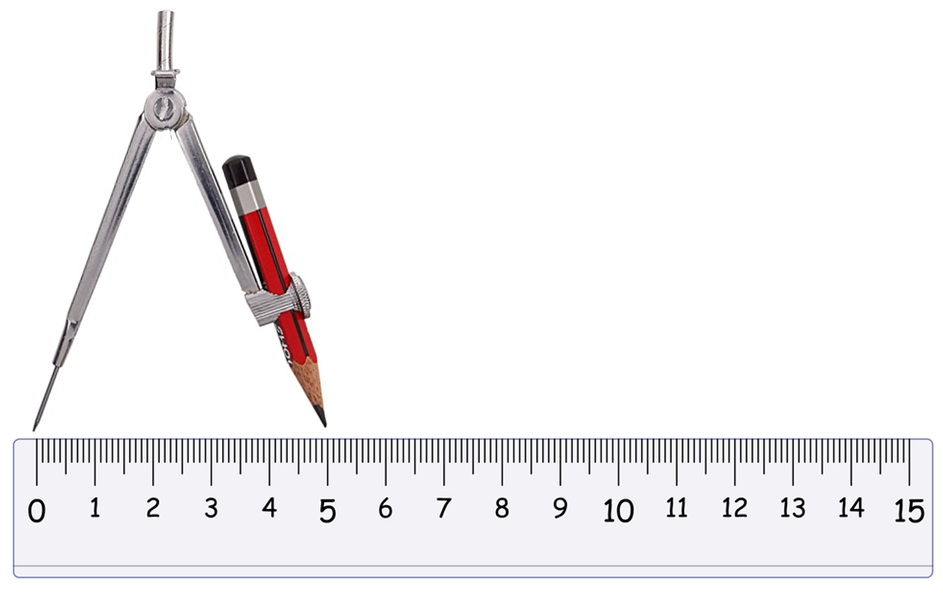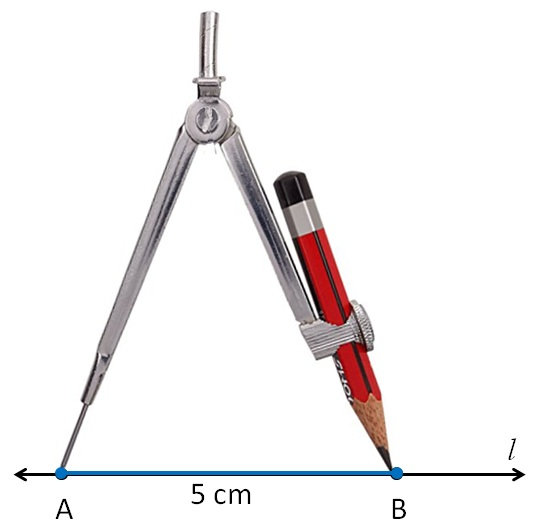Construction of a line segment of a given length

Chapter 14 Class 6 Practical Geometry
Concept wise

Let’s take an example

Draw a line segment of length 5 cm

1. Draw a line l
mark point A on it1. Since length is 5 cm,
we measure 5 cm using ruler and compass2. Now keeping compass opened the same length.

We keep pointed end at point A,

and draw an arc on line lSo, the required line segment is (AB) = 5 cm

Get live Maths 1-on-1 Classs - Class 6 to 12